Open In App

# How to plot Timeseries based charts using Pandas?

A series of data points collected over the course of a time period, and that are time-indexed is known as Time Series data. These observations are recorded at successive equally spaced points in time. For Example, the ECG Signal, EEG Signal, Stock Market, Weather Data, etc., all are time-indexed and recorded over a period of time. Analyzing these data, and predicting future observations has a wider scope of research.

In this article, we will see how to implement EDA — Exploratory Data Analysis using Pandas Library in Python. We will try to infer the nature of the data over a specific period of time by plotting various graphs with matplotlib.pyplot, seaborn, statsmodels, and more packages.

For easy understanding of the plots and other functions, we will be creating a sample dataset with 16 rows and 5 columns which includes Date, A, B, C, D, and E columns.

## Python3

 `import` `pandas as pd` `# Sample data which will be used``# to create the dataframe``sample_timeseries_data ``=` `{` `    ``'Date'``: [``'2020-01-25'``, ``'2020-02-25'``,``             ``'2020-03-25'``, ``'2020-04-25'``,``             ``'2020-05-25'``, ``'2020-06-25'``,``             ``'2020-07-25'``, ``'2020-08-25'``,``             ``'2020-09-25'``, ``'2020-10-25'``,``             ``'2020-11-25'``, ``'2020-12-25'``,``             ``'2021-01-25'``, ``'2021-02-25'``,``             ``'2021-03-25'``, ``'2021-04-25'``],` `    ``'A'``: [``102``, ``114``, ``703``, ``547``,``          ``641``, ``669``, ``897``, ``994``,``          ``1002``, ``974``, ``899``, ``954``,``          ``1105``, ``1189``, ``1100``, ``934``],``  ` `    ``'B'``: [``1029``, ``1178``, ``723``, ``558``,``          ``649``, ``669``, ``899``, ``1000``,``          ``1012``, ``984``, ``918``, ``959``,``          ``1125``, ``1199``, ``1109``, ``954``],``  ` `      ``'C'``: [``634``, ``422``,``152``, ``23``,``         ``294``, ``1452``, ``891``, ``990``,``         ``924``, ``960``, ``874``, ``548``,``         ``174``, ``49``, ``655``, ``914``],``  ` `      ``'D'``: [``1296``, ``7074``, ``3853``, ``4151``,``         ``2061``, ``1478``, ``2061``, ``3853``,``         ``6379``, ``2751``, ``1064``, ``6263``,``         ``2210``, ``6566``, ``3918``, ``1121``],` `    ``'E'``: [``10``, ``17``, ``98``, ``96``,``          ``85``, ``89``, ``90``, ``92``,``          ``86``, ``84``, ``78``, ``73``,``          ``71``, ``65``, ``70``, ``60``]``}` `# Creating a dataframe using pandas``# module with Date, A, B, C, D and E``# as columns.``dataframe ``=` `pd.DataFrame(``  ``sample_timeseries_data,columns``=``[``    ``'Date'``, ``'A'``, ``'B'``, ``'C'``, ``'D'``, ``'E'``])` `# Changing the datatype of Date, from``# Object to datetime64``dataframe[``"Date"``] ``=` `dataframe[``"Date"``].astype(``"datetime64"``)` `# Setting the Date as index``dataframe ``=` `dataframe.set_index(``"Date"``)``dataframe`

Output: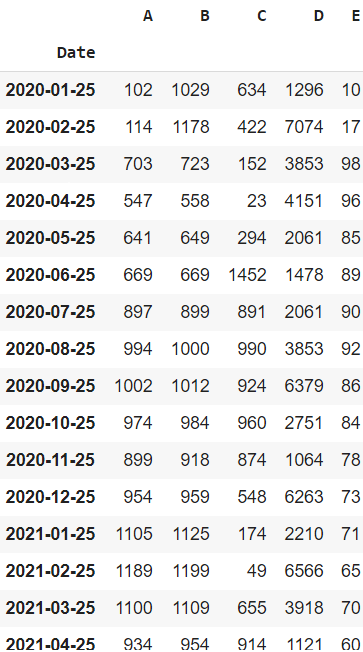Sample Time Series data frame

## Plotting the Time-Series Data

### Plotting Timeseries based Line Chart:

Line charts are used to represent the relation between two data X and Y on a different axis.

Syntax: plt.plot(x)

Example 1: This plot shows the variation of Column A values from Jan 2020 till April 2020. Note that the values have a positive trend overall, but there are ups and downs over the course.

## Python3

 `import` `matplotlib.pyplot as plt` `# Using a inbuilt style to change``# the look and feel of the plot``plt.style.use(``"fivethirtyeight"``)` `# setting figure size to 12, 10``plt.figure(figsize``=``(``12``, ``10``))` `# Labelling the axes and setting``# a title``plt.xlabel(``"Date"``)``plt.ylabel(``"Values"``)``plt.title(``"Sample Time Series Plot"``)` `# plotting the "A" column alone``plt.plot(dataframe[``"A"``])`

Output: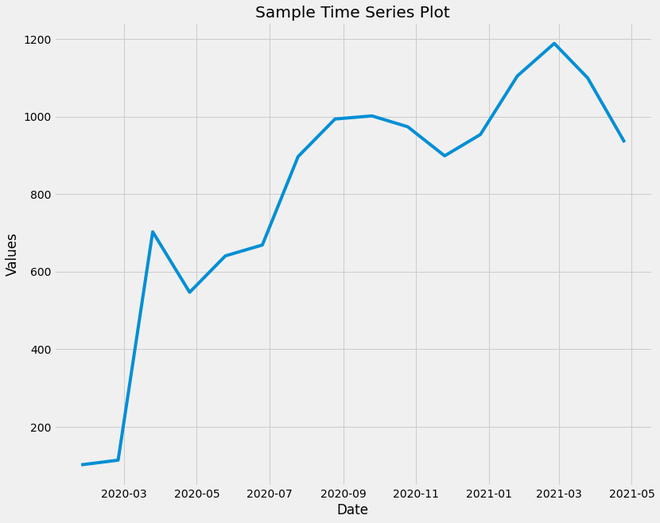Sample Time Series Plot

Example 2: Plotting with all variables.

## Python3

 `plt.style.use(``"fivethirtyeight"``)``dataframe.plot(subplots``=``True``, figsize``=``(``12``, ``15``))`

Output: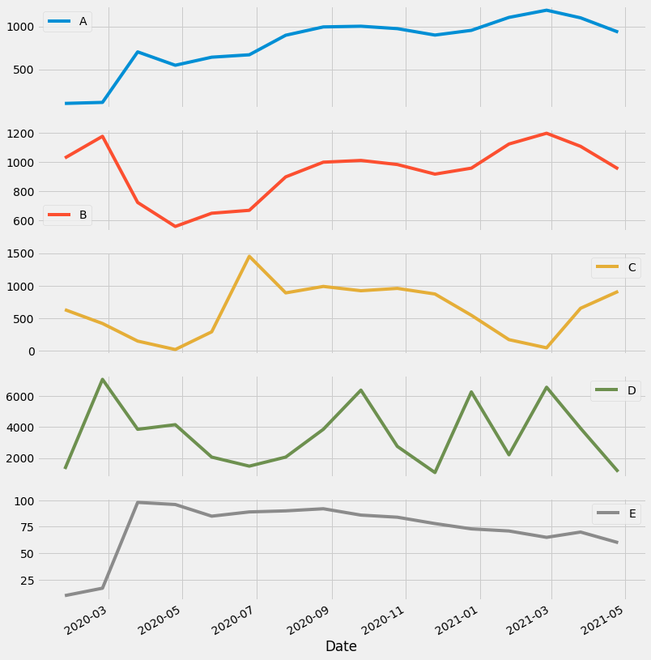Plotting all Time-series data columns

### Plotting Timeseries based Bar Plot:

A bar plot or bar chart is a graph that represents the category of data with rectangular bars with lengths and heights that is proportional to the values which they represent. The bar plots can be plotted horizontally or vertically. A bar chart describes the comparisons between the discrete categories. One of the axis of the plot represents the specific categories being compared, while the other axis represents the measured values corresponding to those categories.

Syntax: plt.bar(x, height, width, bottom, align)

This bar plot represents the variation of the ‘A’ column values. This can be used to compare the future and the fast values.

## Python3

 `import` `matplotlib.pyplot as plt` `# Using a inbuilt style to change``# the look and feel of the plot``plt.style.use(``"fivethirtyeight"``)` `# setting figure size to 12, 10``plt.figure(figsize``=``(``15``, ``10``))` `# Labelling the axes and setting a``# title``plt.xlabel(``"Date"``)``plt.ylabel(``"Values"``)``plt.title(``"Bar Plot of 'A'"``)` `# plotting the "A" column alone``plt.bar(dataframe.index, dataframe[``"A"``], width``=``5``)`

Output:Bar Plot for ‘A’ column

### Plotting Timeseries based Rolling Mean Plots:

The mean of an n-sized window sliding from the beginning to the end of the data frame is known as Rolling Mean. If the window doesn’t have n observations, then NaN is returned.

Syntax: pandas.DataFrame.rolling(n).mean()

Example:

## Python3

 `dataframe.rolling(window ``=` `5``).mean()`

Output: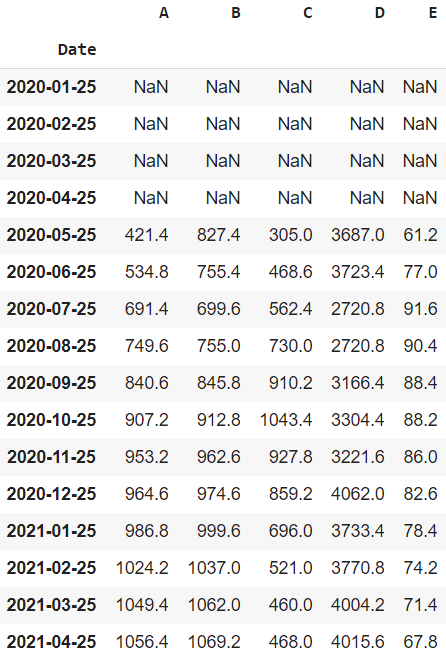The rolling mean of dataframe

Here, we will plot the time series with a rolling means plot:

## Python3

 `import` `matplotlib.pyplot as plt` `# Using a inbuilt style to change``# the look and feel of the plot``plt.style.use(``"fivethirtyeight"``)` `# setting figure size to 12, 10``plt.figure(figsize``=``(``12``, ``10``))` `# Labelling the axes and setting``# a title``plt.xlabel(``"Date"``)``plt.ylabel(``"Values"``)``plt.title(``"Values of 'A' and Rolling Mean (2) Plot"``)` `# plotting the "A" column and "A" column``# of Rolling Dataframe (window_size  = 20)``plt.plot(dataframe[``"A"``])``plt.plot(dataframe.rolling(``  ``window``=``2``, min_periods``=``1``).mean()[``"A"``])`

Output: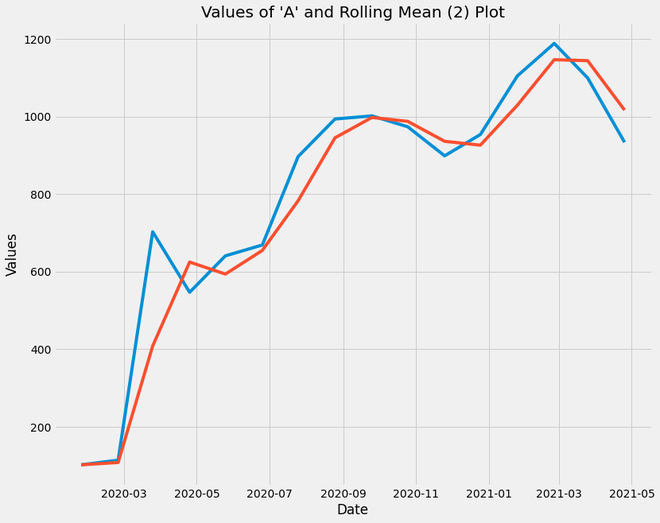Rolling mean plot

Explanation:

• The Blue Plot Line represents the original ‘A’ column values while the Red Plot Line represents the Rolling mean of ‘A’ column values of window size = 2
• Through this plot, we infer that the rolling mean of a time-series data returns values with fewer fluctuations. The trend of the plot is retained but unwanted ups and downs which are of less significance are discarded.
• For plotting the decomposition of time-series data, box plot analysis, etc., it is a good practice to use a rolling mean data frame so that the fluctuations don’t affect the analysis, especially in forecasting the trend.

### Time Series Decomposition:

It shows the observations and these four elements in the same plot:

• Trend Component: It shows the pattern of the data that spans across the various seasonal periods. It represents the variation of ‘A’ values over the period of 2 years with no fluctuations.
• Seasonal Component: This plot shows the ups and downs of the ‘A’ values i.e. the recurring normal variations.
• Residual Component: This is the leftover component after decomposing the ‘A’ values data into Trend and Seasonal Component.
• Observed Component: This trend and a seasonal component can be used to study the data for various purposes.

Example:

## Python3

 `import` `statsmodels.api as sm``from` `pylab ``import` `rcParams``import` `pandas as pd``import` `matplotlib.pyplot as plt``import` `seaborn as sns` `# Separating the Date Component into``# Year and Month``dataframe[``'Date'``] ``=` `dataframe.index``dataframe[``'Year'``] ``=` `dataframe[``'Date'``].dt.year``dataframe[``'Month'``] ``=` `dataframe[``'Date'``].dt.month` `# using inbuilt style``plt.style.use(``"fivethirtyeight"``)` `# Creating a dataframe with "Date" and "A"``# columns only. This dataframe is date indexed``decomposition_dataframe ``=` `dataframe[[``'Date'``, ``'A'``]].copy()``decomposition_dataframe.set_index(``'Date'``, inplace``=``True``)``decomposition_dataframe.index ``=` `pd.to_datetime(decomposition_dataframe.index)` `# using sm.tsa library, we are plotting the``# seasonal decomposition of the "A" column``# Multiplicative Model : Y[t] = T[t] * S[t] * R[t]``decomposition ``=` `sm.tsa.seasonal_decompose(decomposition_dataframe,``                                          ``model``=``'multiplicative'``, freq``=``5``)``decomp ``=` `decomposition.plot()``decomp.suptitle(``'"A" Value Decomposition'``)` `# changing the runtime configuration parameters to``# have a desired plot of desired size, etc``rcParams[``'figure.figsize'``] ``=` `12``, ``10``rcParams[``'axes.labelsize'``] ``=` `12``rcParams[``'ytick.labelsize'``] ``=` `12``rcParams[``'xtick.labelsize'``] ``=` `12`

Output: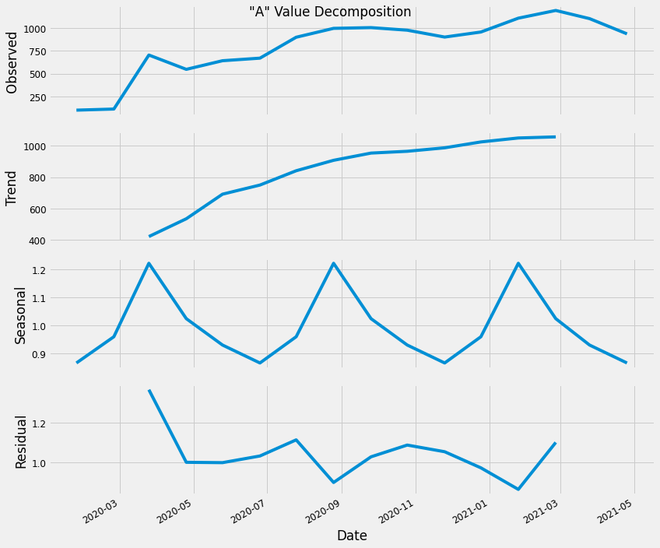‘A’ value decomposition

### Plotting Timeseries based Autocorrelation Plot:

It is a commonly used tool for checking randomness in a data set. This randomness is ascertained by computing autocorrelation for data values at varying time lags. It shows the properties of a type of data known as a time series. These plots are available in most general-purpose statistical software programs. It can be plotted using the pandas.plotting.autocorrelation_plot().

Syntax: pandas.plotting.autocorrelation_plot(series, ax=None, **kwargs)

Parameters:

• series: This parameter is the Time series to be used to plot.
• ax: This parameter is a matplotlib axes object. Its default value is None.

Returns: This function returns an object of class matplotlib.axis.Axes

Considering the trend, seasonality, cyclic and residual, this plot shows the current value of the time-series data is related to the previous values. We can see that a significant proportion of the line shows an effective correlation with time, and we can use such correlation plots to study the internal dependence of time-series data.

Code:

## Python3

 `from` `pandas.plotting ``import` `autocorrelation_plot` `autocorrelation_plot(dataframe[``'A'``])`

Output: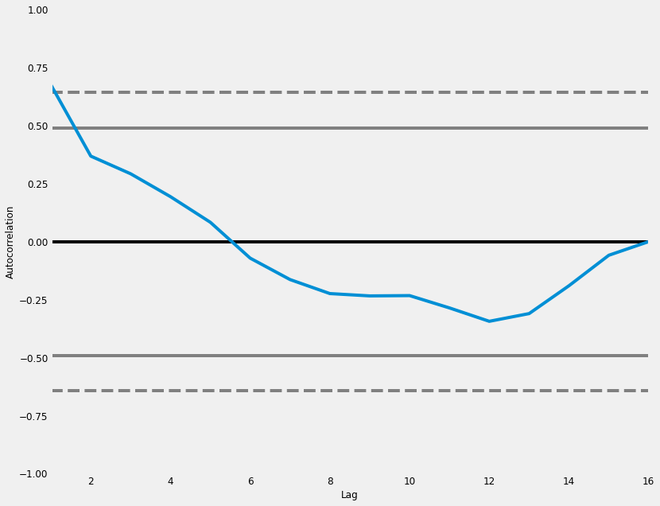Autocorrelation plot

### Plotting Timeseries based Box Plot:

Box Plot is the visual representation of the depicting groups of numerical data through their quartiles. Boxplot is also used for detecting the outlier in data set. It captures the summary of the data efficiently with a simple box and whiskers and allows us to compare easily across groups. Boxplot summarizes a sample data using 25th, 50th and 75th percentiles.

Syntax: seaborn.boxplot(x=None, y=None, hue=None, data=None, order=None, hue_order=None, orient=None, color=None, palette=None, saturation=0.75, width=0.8, dodge=True, fliersize=5, linewidth=None, whis=1.5, ax=None, **kwargs)

Parameters:
x, y, hue: Inputs for plotting long-form data.
data: Dataset for plotting. If x and y are absent, this is interpreted as wide-form.
color: Color for all of the elements.

Returns: It returns the Axes object with the plot drawn onto it.

Here, through these plots, we will be able to obtain an intuition of the ‘A’ value ranges of each year (Year-wise Box Plot) as well as each month (Month-wise Box Plot). Also, through the Month-wise Box Plot, we can observe that the value range is slightly higher in Jan and Feb, compared to other months.

## Python3

 `# Splitting the plot into (1,2) subplots``# and initializing them using fig and ax``# variables``fig, ax ``=` `plt.subplots(nrows``=``1``, ncols``=``2``,``                       ``figsize``=``(``15``, ``6``))` `# Using Seaborn Library for Box Plot``sns.boxplot(dataframe[``'Year'``],``            ``dataframe[``"A"``], ax``=``ax[``0``])` `# Defining the title and axes names``ax[``0``].set_title(``'Year-wise Box Plot for A'``,``                ``fontsize``=``20``, loc``=``'center'``)``ax[``0``].set_xlabel(``'Year'``)``ax[``0``].set_ylabel(``'"A" values'``)` `# Using Seaborn Library for Box Plot``sns.boxplot(dataframe[``'Month'``],``            ``dataframe[``"A"``], ax``=``ax[``1``])` `# Defining the title and axes names``ax[``1``].set_title(``'Month-wise Box Plot for A'``,``                ``fontsize``=``20``, loc``=``'center'``)``ax[``1``].set_xlabel(``'Month'``)``ax[``1``].set_ylabel(``'"A" values'``)` `# rotate the ticks and right align them``fig.autofmt_xdate()`

Output: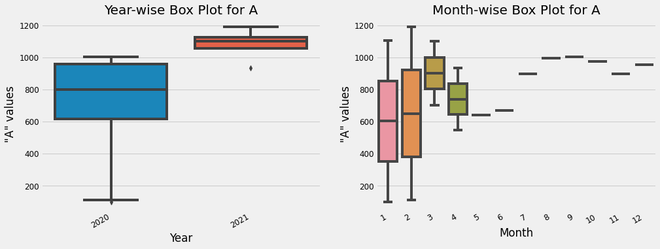box plot analysis of ‘A’ column values

### Shift Analysis:

This plot the achieved by dividing the current value of the ‘A’ column by the shifted value of the ‘A’ column. Default Shift is by one value. This plot is used to analyze the value stability on a daily basis.

## Python3

 `dataframe[``'Change'``] ``=` `dataframe.A.div(dataframe.A.shift())``dataframe[``'Change'``].plot(figsize``=``(``15``, ``10``),``                         ``xlabel ``=` `"Date"``,``                         ``ylabel ``=` `"Value Difference"``,``                         ``title ``=` `"Shift Plot"``)`

Output: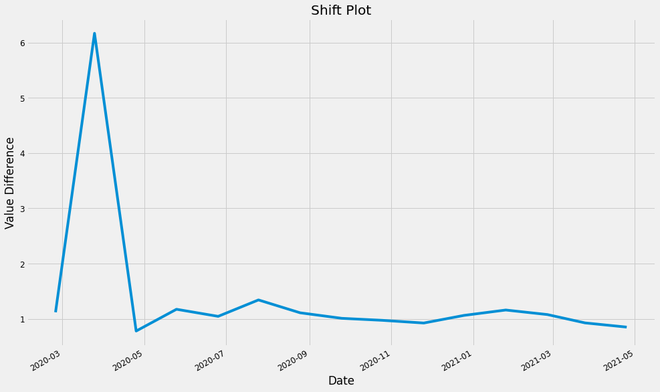shift plot of the ‘A’ values

### Plotting Timeseries based Heatmap:

We can interpret the trend of the “A” column values across the years sampled over 12 months, variation of values across different years, etc. We can also infer how the values have changed from the average value. This heatmap is a really useful visualization. This Heatmap shows the variation of temperature across Years as well as Months, differentiated using a Colormap.

## Python3

 `import` `calendar``import` `seaborn as sns``import` `pandas as pd` `dataframe[``'Date'``] ``=` `dataframe.index` `# Splitting the Date into Year and Month``dataframe[``'Year'``] ``=` `dataframe[``'Date'``].dt.year``dataframe[``'Month'``] ``=` `dataframe[``'Date'``].dt.month` `# Creating a Pivot Table with "A"``# column values and is Month indexed.``table_df ``=` `pd.pivot_table(dataframe, values``=``[``"A"``],``                                   ``index``=``[``"Month"``],``                                   ``columns``=``[``"Year"``],``                                   ``fill_value``=``0``,``                                   ``margins``=``True``)` `# Naming the index, can be generated``# using calendar.month_abbr[i]``mon_name ``=` `[[``'Jan'``, ``'Feb'``, ``'Mar'``, ``'Apr'``,``             ``'May'``, ``'Jun'``, ``'Jul'``, ``'Aug'``,``             ``'Sep'``,``'Oct'``, ``'Nov'``, ``'Dec'``, ``'All'``]]` `# Indexing using Month Names``table_df ``=` `table_df.set_index(mon_name)` `# Creating a heatmap using sns with Red,``# Yellow & Green Colormap.``ax ``=` `sns.heatmap(table_df, cmap``=``'RdYlGn_r'``,``                 ``robust``=``True``, fmt``=``'.2f'``,``                 ``annot``=``True``, linewidths``=``.``6``,``                 ``annot_kws``=``{``'size'``:``10``},``                 ``cbar_kws``=``{``'shrink'``:.``5``,``                           ``'label'``:``'"A" values'``})                      ` `# Setting the Tick Labels, Title and x & Y labels``ax.set_yticklabels(ax.get_yticklabels())``ax.set_xticklabels(ax.get_xticklabels())``plt.title(``'"A" Value Analysis'``, pad``=``14``)``plt.xlabel(``'Year'``)``plt.ylabel(``'Months'``)`

Output: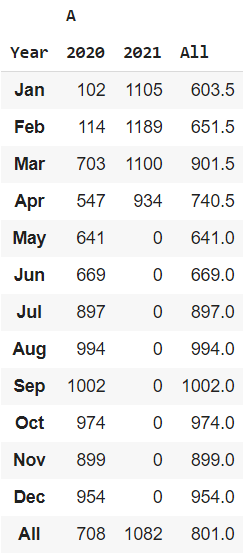heatmap table for “A” column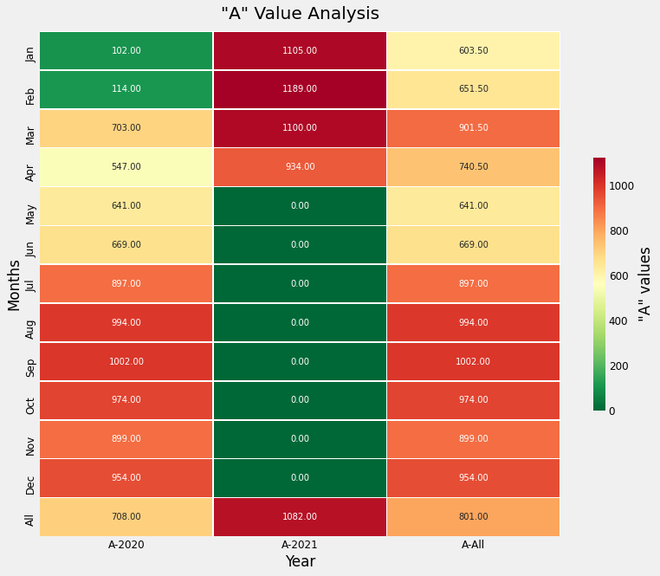Heatmap Plot for the “A” column values